# Reverb音效算法研究实现

1. Reverb物理原型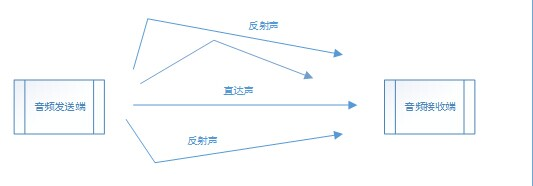图1. 音频信号传输

2. 数字信号处理模型

2.1 梳状滤波器模型

y(n) = x(n) + ax(n-m) + a2 x(n-2m) + ……

H(z) = 1+a z-m + a2 z-2m +……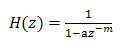y(n) = ay(n-m) + x(n)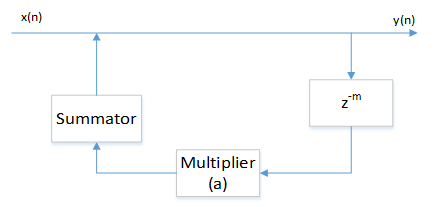图2. 梳状滤波器系统框图

2.2 全通滤波器模型

y(n) = -gx(n) + x(n-m) +gy(n-m);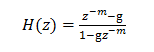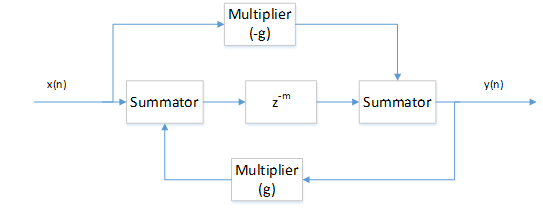2.3 Schroeder混合模型
综合梳状滤波器和全通滤波器，为了实现较高的回声密度，将4个梳状滤波器进行并联，然后通过2个级联的全通滤波器，实现Schroder混合模型。Schroeder混合模型的系统框图如图4所示。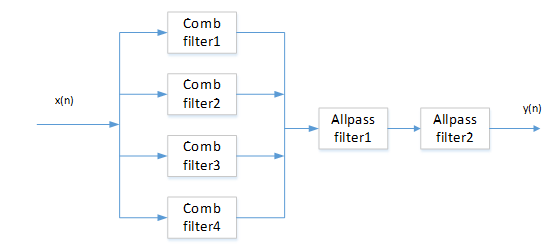3.  算法实现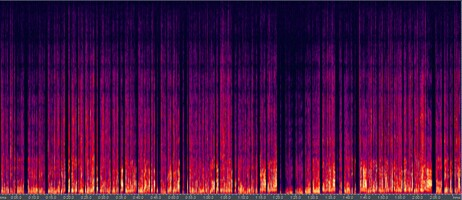(a) 原始音频文件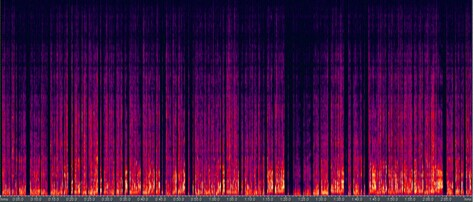(b) 小房间模型数据